GFG App
Open AppBrowser
Continue

# How to Create a Relative File Path with Power Query in Excel?

A path is a unique location for a file or a folder. There are broadly two types of paths, absolute path, and relative path. An absolute path gives the location of a file from its root folder, while a relative path gives the location of a file from its current folder. Power Query, by default, provides an absolute path, which could cause problems while sharing the files, so we have to reduce the absolute path to a relative path. In this article, we will learn how to convert an absolute path to a relative path, using a power query editor, and make our source files sharable.

## Problem with Absolute Path

By default, our excel sheets have the location for absolute paths and not the relative paths. Due, to this file sharing, is a big and significant issue. For example, an analyst is analyzing data in its intelligence tools source via power query from your PC, the tools work well until the files are in your PC, but stop working as soon as they are imported into the third person PC, this happens because the absolute path in the third person computer might be different, and our power query files are still searching for the old absolute path provided in my computer. To resolve this issue, one needs to convert this absolute path to the relative path, so that the files can access irrespective of one’s PC.

## Excel Functions Used in Converting Absolute Path to Relative Path

Before moving forward, we need to have a crisp knowledge of all the excel functions, that will be used to convert an Absolute path to a Relative path. There are broadly three functions used: =CELL(), =LEFT(), =FIND(), for creating the required formula:

### Cell Function

Syntax: =CELL(info_type, reference)

The cell function provides every information, you require, for a cell. One can get the value of a cell, its row number, address, filepath, etc. This topic could be quite big itself, but for converting absolute path to relative path, we only need to know about how to get the file path of a cell. There are two arguments in cell functions:

• Argument 1: Info_type is the first argument of cell function. The type of information you want to find for the specified cell. For example, “filepath”, provides the absolute path for the current cell.
• Argument 2: For which cell, do you want to extract the information. The cell reference can be absolute or relative.

Note: The excel file should be saved in some folder, then only the absolute path would appear, otherwise it will show an empty string returned. This is one of the common errors that users face while working with the =CELL(info_type, reference) function.

For example, find the absolute path of the current opened excel file. Following are the steps:

Step 1: Type =CELL(“filename”, \$A\$1), in cell B2, where “filename” provides the absolute reference of the file, and \$A\$1 is the reference to cell A1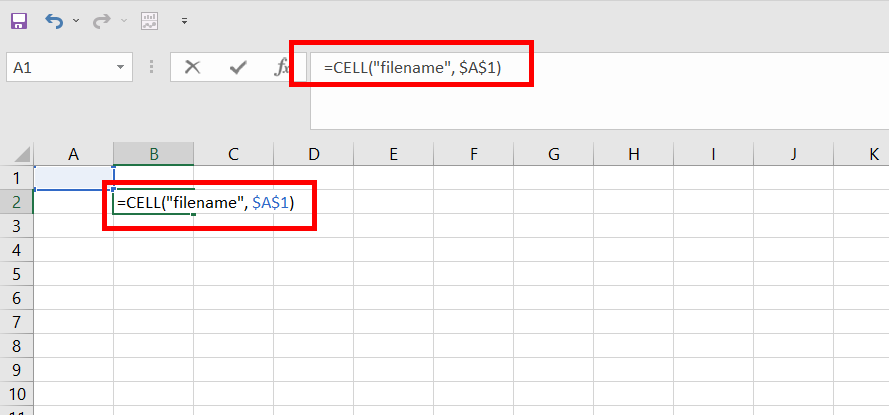Step 2: Press Enter. The absolute Path of the current excel file appears in cell B2 i.e. D:\geeksforgeeks\folder1\folder2\[geeksample.xlsx]Sheet1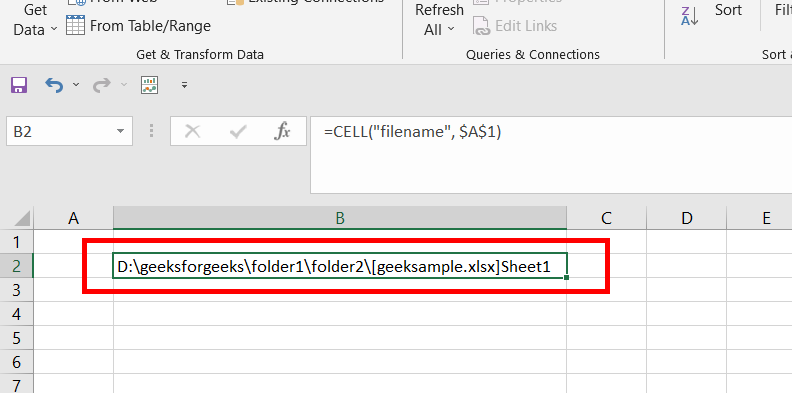Step 3: The path before the bracket is the absolute path for that excel file. The text inside the square brackets is the name of the workbook, and at last, is the name of the worksheet.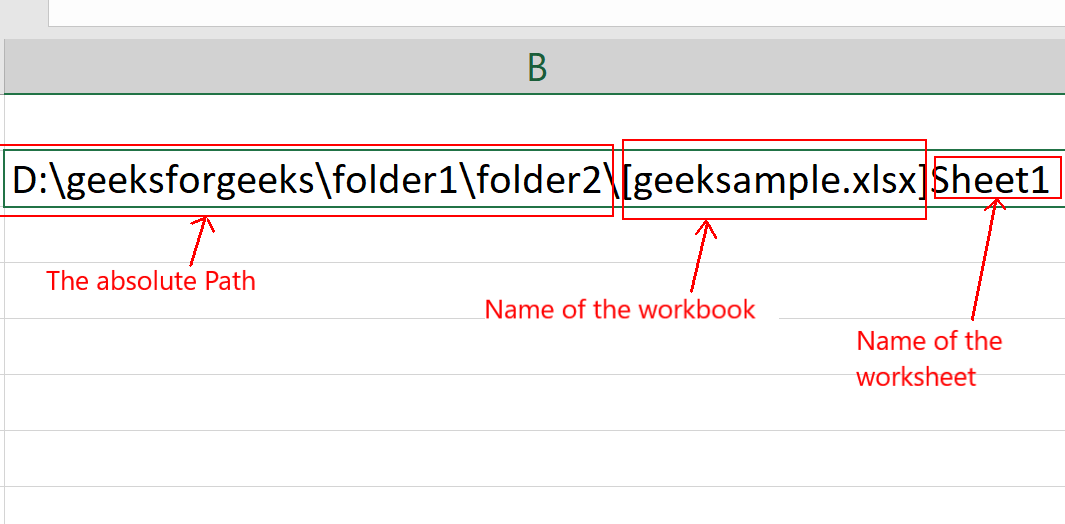### Find Function

Syntax: =FIND(find_text, within_text, [start_num])

The function finds the first starting index of the location of a finding text in the given string. The indexing is 1-based. For example, if you are given a string “geeksforgeeks”, and you want to find the position of “ks” in your given string, then the answer returned by the =FIND() function will be 4. There are three arguments in the find function, but for converting the absolute path to a relative path, we will require only the first two arguments.

• Argument 1: Find_text is the first argument of the find function. The find_text is the string that needs to be found in the given string. The first occurrence of the find_text is printed using the =FIND() function.
• Argument 2: Within_text is the second argument of the find function. The within_text is the original string in which find_text is searched.
• Argument 3: This is an optional argument. It tells from which index you should start the search in the within_text.

For example, you recently found the absolute path for the current excel file, our task is to find the index of “[” (square bracket) in the given string i.e. “D:\geeksforgeeks\folder1\folder2\[geeksample.xlsx]Sheet1”. Following are the steps:

Step 1: Type =FIND(“[“, “D:\geeksforgeeks\folder1\folder2\[geeksample.xlsx]Sheet1”), in the cell B2, where Argument1 is “[“ and Argument2 is “D:\geeksforgeeks\folder1\folder2\[geeksample.xlsx]Sheet1”. Press Enter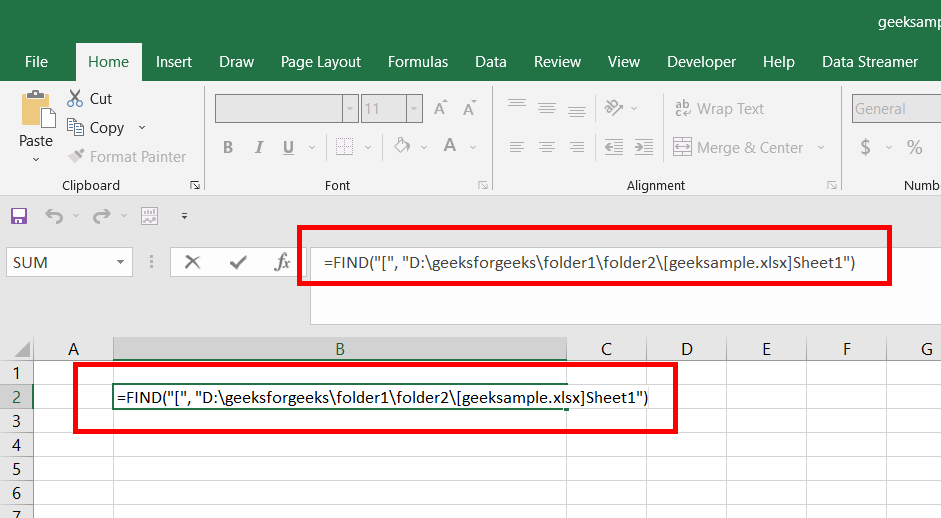Step 2: We can see 34 appear in cell B2. This is because the “[“ appears at the 34th (1-based indexing) index in the original string.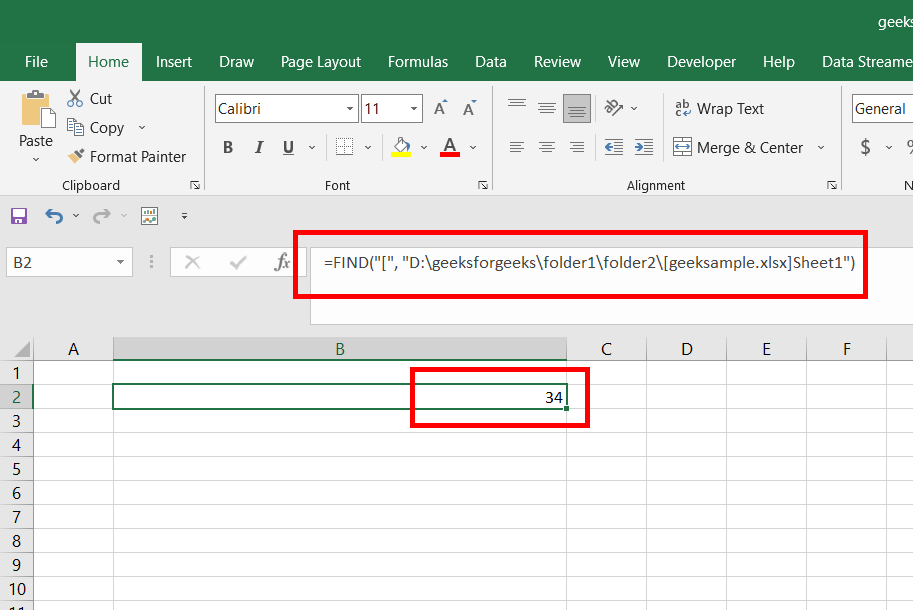### Left Function

Syntax: =LEFT(text, [num_chars])

The function returns the prefix substring of a string, according to the user-specified number of characters. The indexing is 1-based. For example, if you are given a string “geeksforgeeks”, and you want to find the first four(4) characters in the given string, then we can use the =LEFT() function, to achieve this, the function will return a prefix substring “geek”. There are two arguments in the left function.

• Argument 1: The first argument is the text string. The text string is the string for which the prefix substring has to be returned.
• Argument 2: The second argument is num_chars. The num_chars is the number of characters you want from the starting of the text string.

For example, you recently found the absolute path of the current worksheet, and you also found the index of the square bracket “[” i.e. 34, our task is to find the prefix substring before the “[” (square bracket) which means the number of character to be 33. Following are the steps:

Step 1: Type =LEFT(“D:\geeksforgeeks\folder1\folder2\[geeksample.xlsx]Sheet1”, 33), in the cell B2, where argument1 is the original string, for which prefix substring has to be returned, and the second argument is the number of characters for which this given string has to be returned.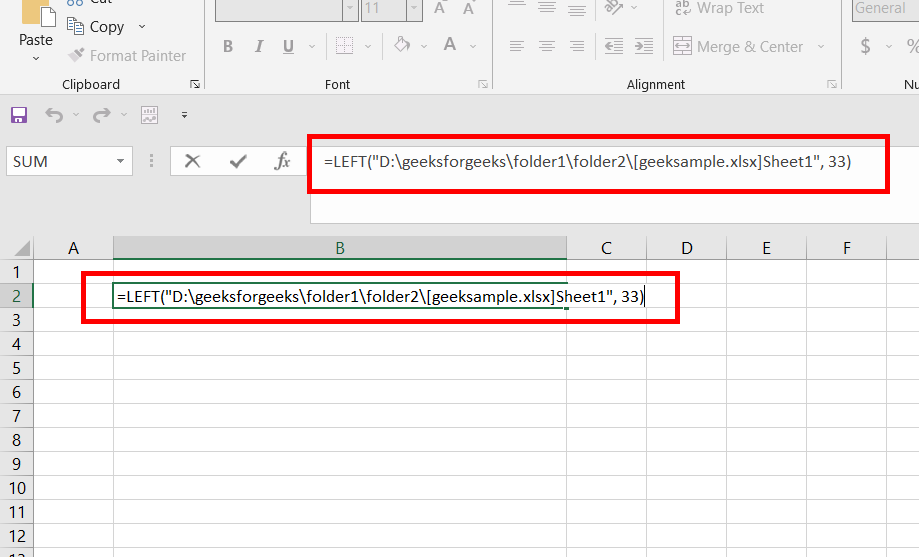Step 2: Press Enter. All first 33 characters will appear in cell B2 i.e. “D:\geeksforgeeks\folder1\folder2\”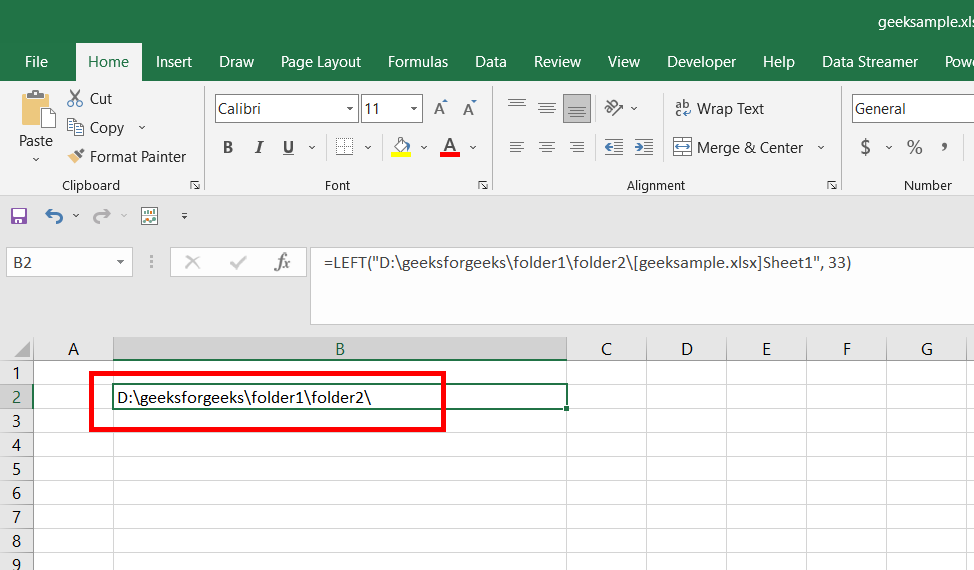### Converting Absolute Path to Relative Path in Power Query

Now, you know everything to convert an absolute path to a relative path, in a power query. We will take the same example and file location which we did, for understanding the functions. The absolute path for the current file is “D:\geeksforgeeks\folder1\folder2\housing.csv”.This needs to be converted into the relative path. Following are the steps:

Step 1: As you are understanding an advanced topic of power query, so we will assume that you know how to get data from a CSV or xlsx file in power query. Imported a table, in the current sheet name “housing”, using Get Data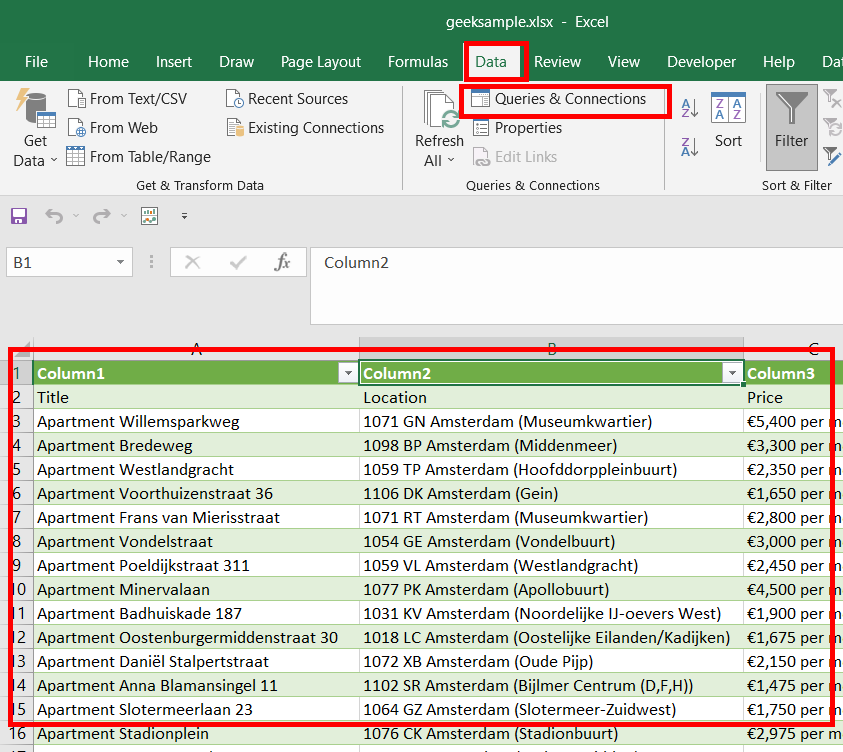Step 2: Now, on the right side of your screen, you will have the housing data set, that you imported. Double-click on it and the power query editor will be launched. The main aim for step1 and step2 is to open our power query editor.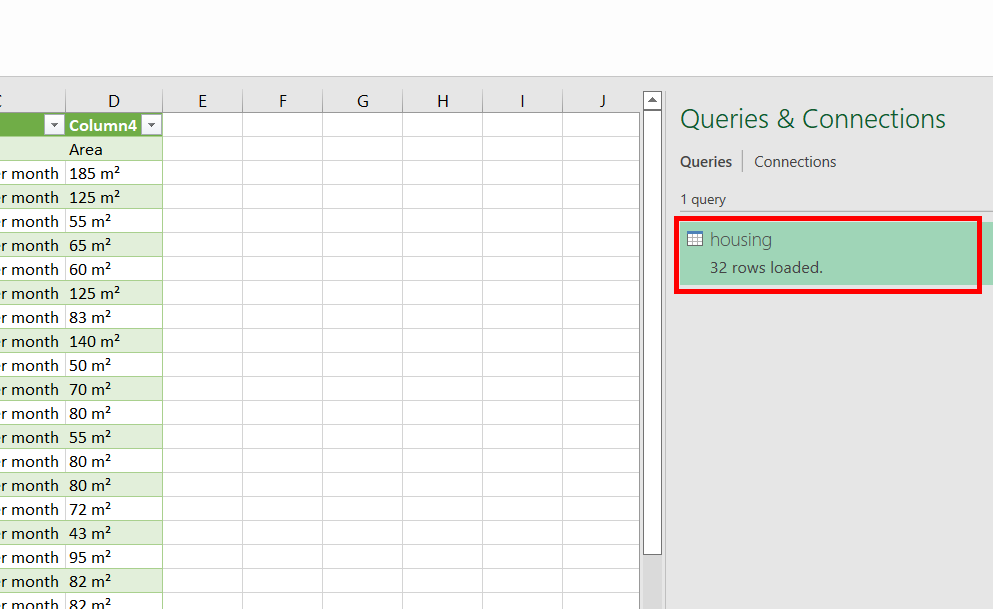Step 3: The power Query editor is opened. In the Home tab, under the Query section, click on Advanced Editor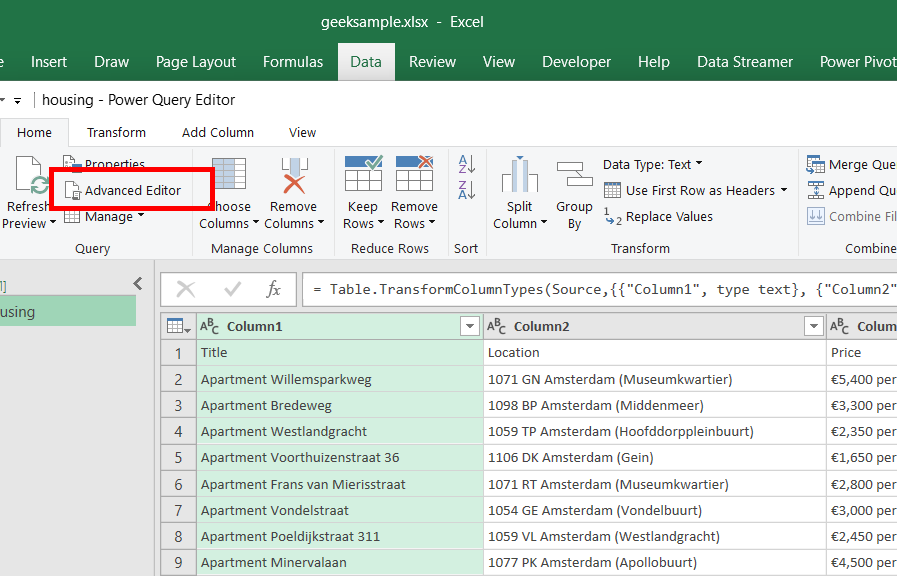Step 4: The Advanced Editor is opened. You can observe that the M-code is written in the picture shown below, and the absolute path for the current CSV file is  “D:\geeksforgeeks\folder1\folder2\housing.csv”. Our task is to convert this absolute path into a relative path.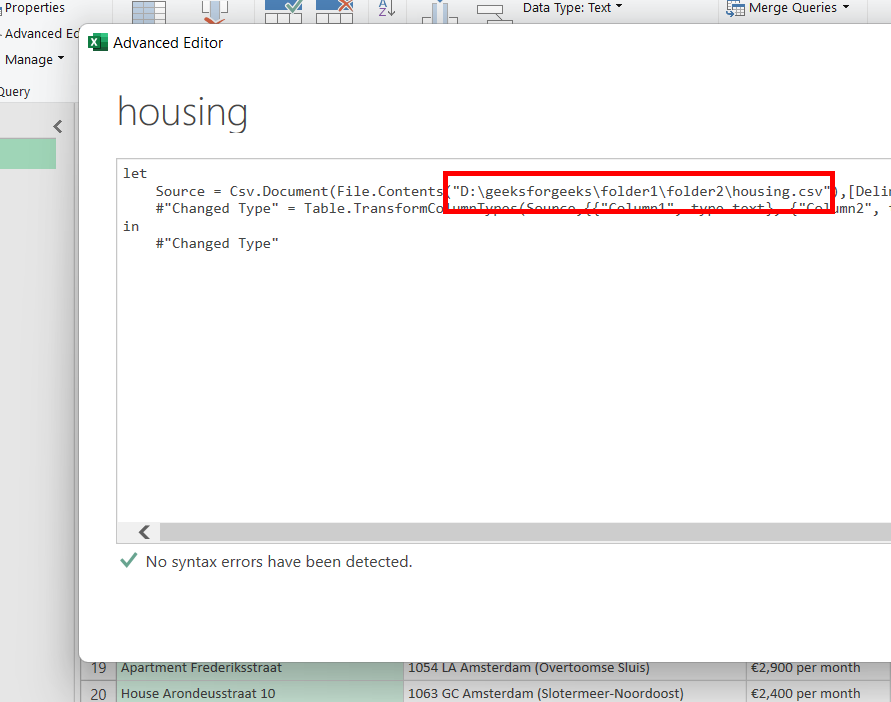Step 5: Close all the windows, and return back to the “housing” worksheet. Now, create a new worksheet, named “setUp”. Cell B2 has the value FilePath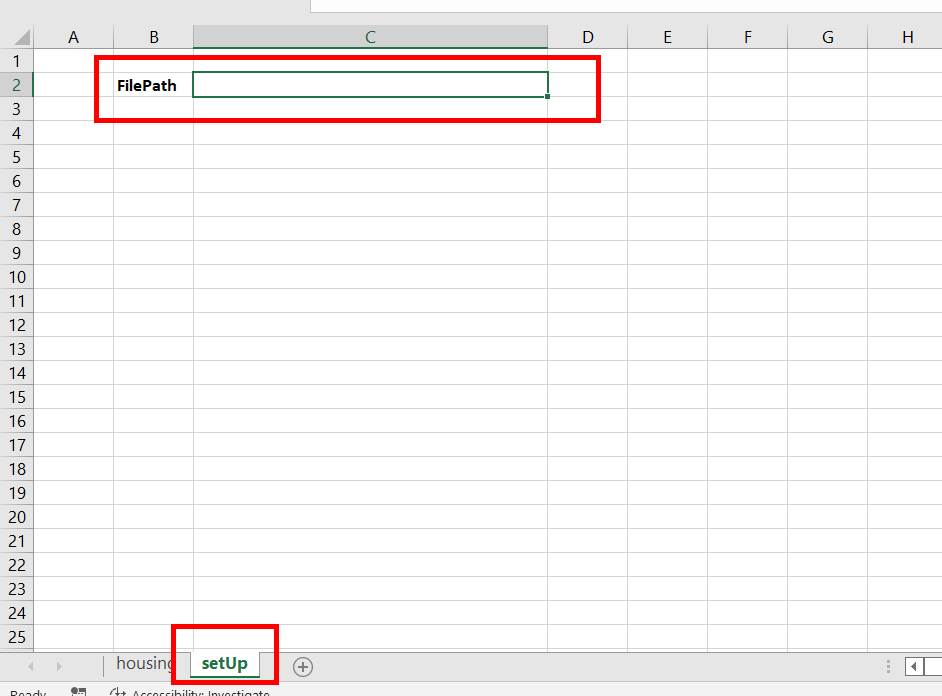Step 6: Now, comes the most important step. Now, if you have understood the examples provided while explaining the different functions like =CELL(), =FIND(), =LEFT(), then this will be a very easy step for you. The formula written is: =LEFT(CELL(“filename”, \$A\$1), FIND(“[“, CELL(“filename”, \$A\$1)) – 1). The formula simply extracts the text written before the square bracket “[“, in the absolute path, i.e. from “D:\geeksforgeeks\folder1\folder2\[geeksample.xlsx]setUp” to “D:\geeksforgeeks\folder1\folder2\”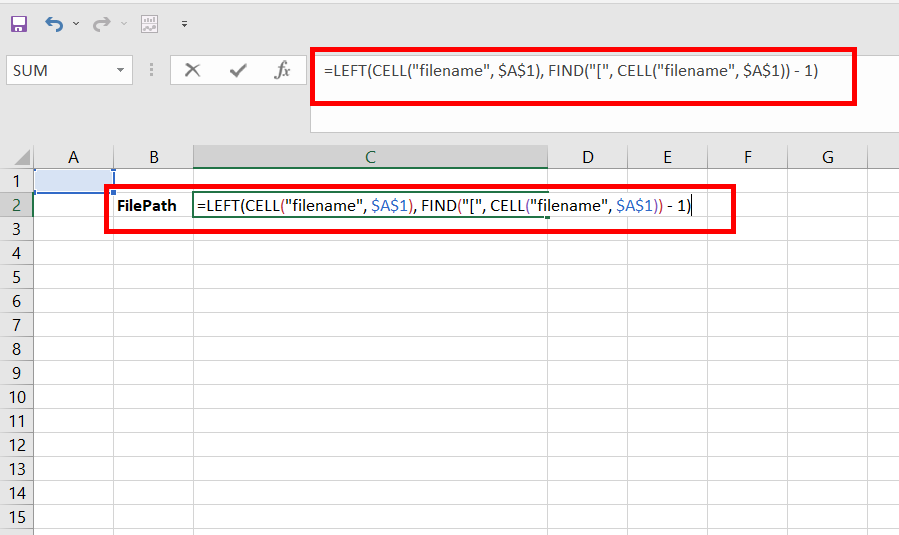Step 7:  Press Enter. “D:\geeksforgeeks\folder1\folder2\” appears in the cell C2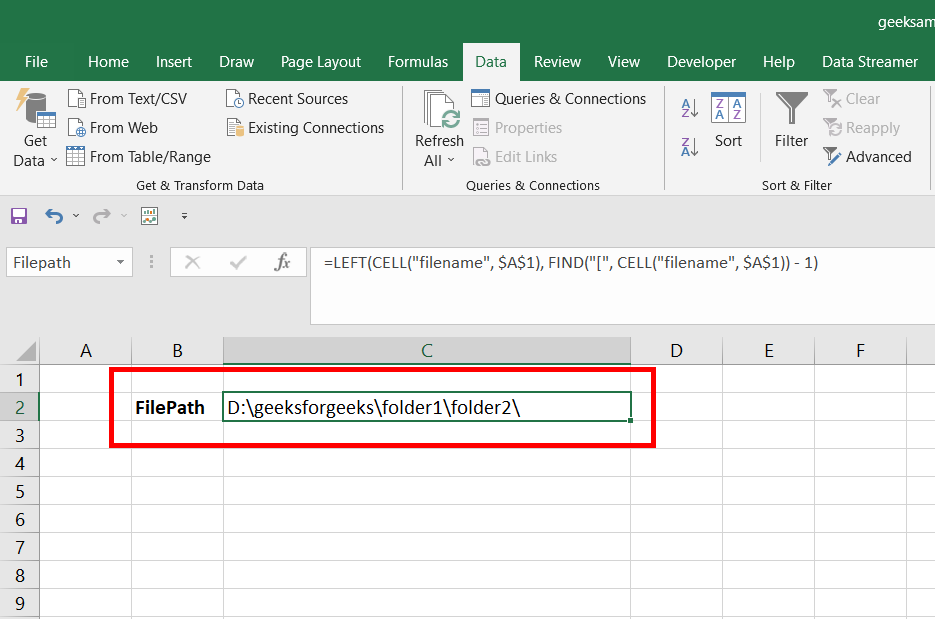Step 8: Change the name of the cell, C3 to Filepath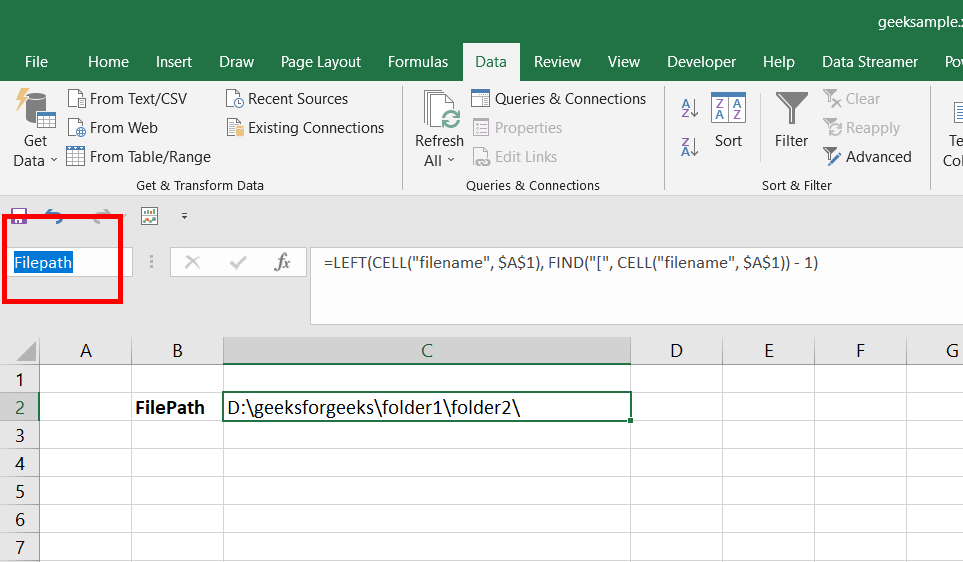Step 9: Now, repeat steps 1, 2, and 3. This will open our advance editor again. Now, add this single line of M-code in your advanced editor, “Filepath = Excel.CurrentWorkbook(){[Name=”Filepath”]}[Content]{0}[Column1],”. This code simply stores the reference of the cell C3(Filepath) in the variable “Filepath”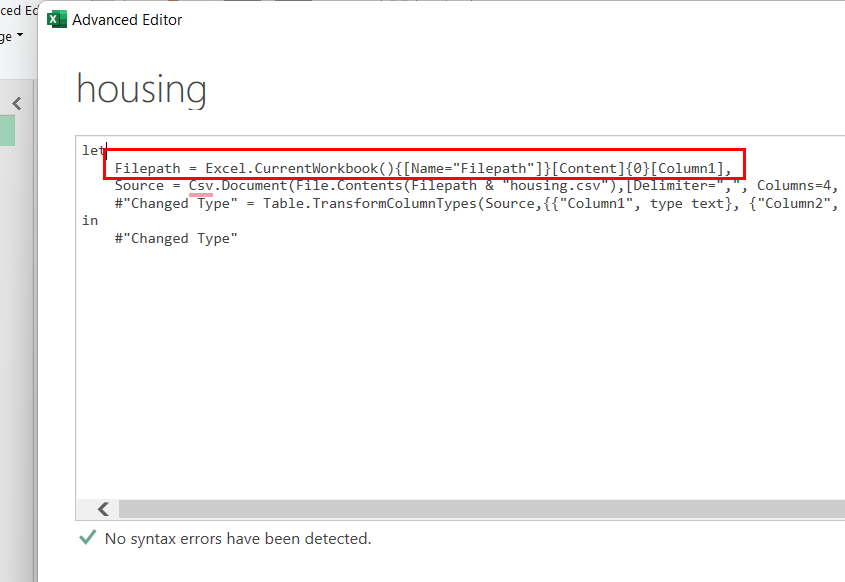Step 10: Now, come to the second line of M-code, where some File content is stored in the variable “Source”. Inside the File.Contents function, change “D:\geeksforgeeks\folder1\folder2\housing.csv” to ” Filepath & “housing.csv” “. Close all the windows, and return back to the “housing” worksheet, this converts your absolute path to the relative path of the current added data in your worksheet.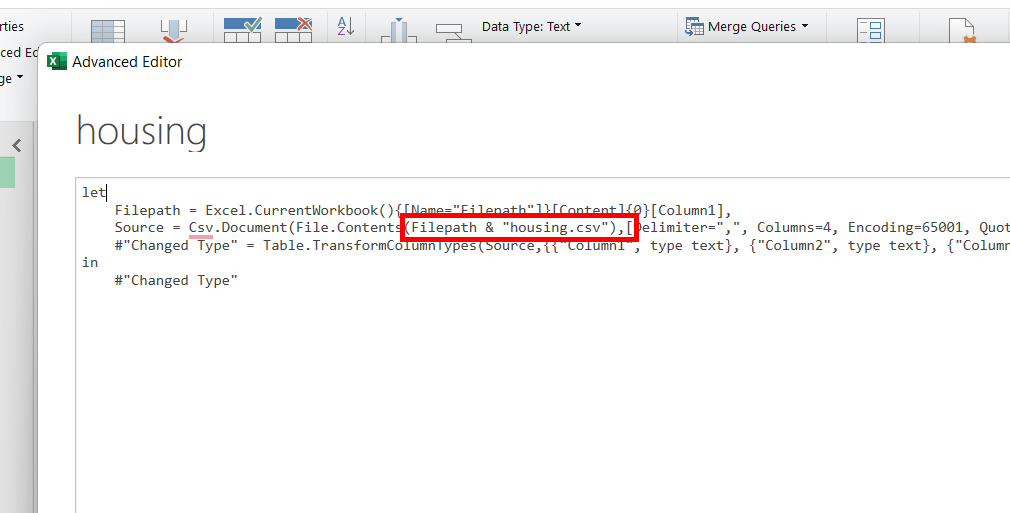My Personal Notes arrow_drop_up